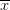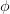##### Question

In: Civil Engineering

# Select double angle tension member to resist a factored load of 100 kips. Member is connected...

Select double angle tension member to resist a factored load of 100 kips. Member is connected with 2 lines of 3⁄4-inch diameter bolts placed at usual gage distances (see AISC manual specification J3.3). There will be more than 2 bolts in each line. Member is 15’-0” long. Hint: Try an angle with 6 inch legs back to back and an outstanding length of 3 1⁄2 -inches. Choose required thickness. Assume U=0.85 for design.

## Solutions

##### Expert Solution

Ans) Given,

Diameter of bolts = 3/4"

Yield strength of steel(fy) = 50 ksi

Ultimate strength of steel(fu) = 65 ksi

Since, it is given that number of bolts in a line should be more then 2 so assuming 3 bolt in a line.

Using angle of 6" x 6" x 5/16" having properties as follows:

A = 3.65 in2 , weight = 14.9 lb/ft

I =13 in4 ,= 1.616 in

Required tensile strength = 100kips

Strength for 2 angles (double angle) = 2 fy A

= 2 x 50 x 3.65

= 365 kips > 100kips

Here ,assuming stress reduction factor = 0.90

Hence stength = 0.9 x 365

= 328.5 kips > 100kips

Available tensile rupture strength(Pn) ,

Pn = Fu x Ae

Ae = U x An

An = A - 2(Db + 1/18" ) t

Putting values,

An = (2x3.65) - 2(3/4 + 1/8)5/16

= 7.3 - 0.546

= 6.753 in2

Therefore, Ae = 0.85 x 6.753

= 5.74 in2

Hence, Pn = 65 x 5.74

= 373.1 kips

Strength reduction factor() = 0.75,

Hence,Pn =  0.75 x 373.1

= 279.825 kips > 100 kips

Also , we know,

U = 1 -/ (n x s) , where n is number of bolts and s is spacing

0.85 = 1 - 1.616/(3 x s)

Therefore, s = 3.6 in

where, n = number of bolts

s = spacing of bolts

Hence, use two 6" x 6" x 5/16" angle section and 3/4" diameter of bolts @ 3.6"

## Related Solutions

##### A 100 ohms load is connected to a 50 ohms transmission line. Determine the value of...
A 100 ohms load is connected to a 50 ohms transmission line. Determine the value of the reactance to put in series at the input to the line and the length in wavelengths of the shortest transmission line to match with a 50 ohms source.
##### The rigid structural member is subjected to a couple consisting of the two 100-N forces. Replace this couple by an equivalent couple consisting of the two forces P and -P, each of which has a magnitude of 400 N. Determine the proper angle θ.
The rigid structural member is subjected to a couple consisting of the two 100-N forces. Replace this couple by an equivalent couple consisting of the two forces P and -P, each of which has a magnitude of 400 N. Determine the proper angle θ.